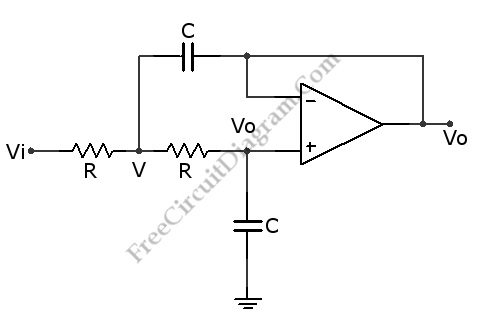# Sallen and Key Lowpass FilterThis a Sallen and Key Lowpass Filter circuit. This circuit is a second-order active filter. This circuit can be changed to highpass filter by interchanging capacitors and resistors. This circuit is featured with buffer. The buffer of this circuit is op-amp which configured in inverting configuration. This circuit is stable, since this circuit has positive feedback and never overcomes the negative feedback. Here is the schematic diagram of the circuit:This circuit uses 411 as op-amp that allow the output and input to swing around ground. The capacitor impedance is 1/jωC, so V is determined by following equation:V = Vo + jωCVoR = Vo(1 + jωCR) and Vi = V + [jωCVo + jωC(V – Vo)] = V(1 + jωCR). So the gain of this circuit is Vo/Vi = 1 / square((1 + jωCR)). There is a square of frequency in the denominator which cause this circuit give second order response.

The gain of this circuit is 40 dB per decade or vary as square(f) if this circuit used at high enough frequency. This gain is twice the slope of a simple RC filter. The pole frequency of this circuit is fo = 1/2ωRC and The tangent of the phase angle is -2(f/fo) / (1 – square(f/fo)). at high frequencies, the phase angle will lead by 180